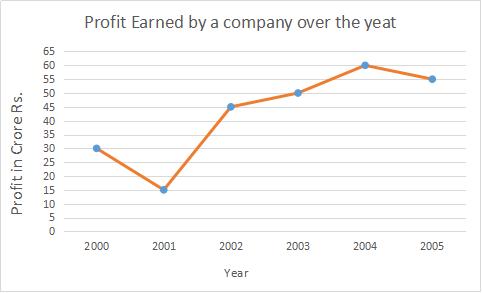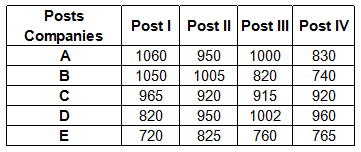## IBPS Quant Test 19

Instructions

Study the following table carefully and answer the questions given below :
Number of Candidates Applying for Various Posts Across Various CompaniesQ 1

The total number of candidates applying for various posts in Company E are what per cent of the total number of candidates applying for various posts in Company A ? (Rounded off to two digits after decimal)

Q 2

The number of candidates applying for Post. III in Company E are approximately what per cent of the total number of candidates applying for the various posts in the same Company?

Q 3

What is the difference between the average number of candidates applying for Post II and the average number of candidates applying for Post IV across various Companies?

Q 4

What is the respective ratio of the total number of candidates applying for various posts in Company A to the total number of candidates
applying for various posts in Company C?

Q 5

What is the approximate average number of candidates applying for Post III in various companies ?